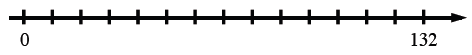### Home > MC1 > Chapter 4 > Lesson 4.1.3 > Problem4-34

4-34.

If a set of $xy\text{‑axes}$ has $20$ grid units in each direction, and the greatest value of the data to be placed on the horizontal axis is $132$, what would be an appropriate scale to use for this axis? Explain.

It may help to draw the situation. See below.Would making each interval $\frac{132}{20}$ be appropriate?

Since $\frac{132}{20}=6.6$, the best interval would be $7$